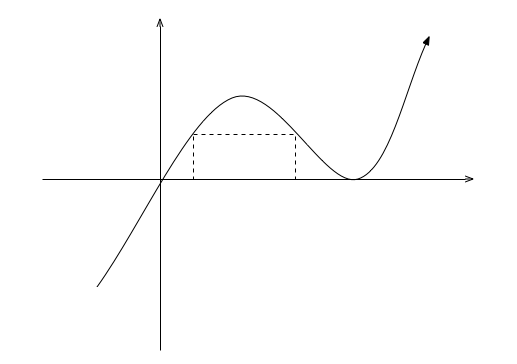Calculus

# Extrema: Level 5 ChallengesBenito the Bandit is hiding out in the center of a large circular lake of radius $30$ miles. He has access to a speedboat, which can travel at a maximum speed of $30$ miles per hour.

Police officer Parker arrived in his Chevrolet Impala police cruiser, with a court order to capture Benito the Bandit. He is a single officer, and is unable to drive (or swim, or fly) over the lake. He figures that the best way to capture Benito is to always drive towards the point on the shore which is closest to Benito.

What is the minimum integer speed at which Officer Parker would have to be able to drive, in order to be certain of catching Benito right when Benito reaches the shore?What is the maximum fractional coverage of the area of a circle by three, non-overlapping rectangles, all of which lie internal to the circle? (The rectangles can be of different sizes.)

If the maximum is $S$, then enter $\lceil 1000*S \rceil$ as your answer.

What is the minimum value that the expression

$\dfrac{x^2y^2 - 2 x^2y +2x^2 +2xy-2x+1}{x^2y + x}$

when $x,y \in \mathbb{R^+}$, can attain? Express your answer to four decimal places.

A rectangle's bottom is at $y=0$while its top corners are on the curve $y=x{ (x-1) }^{ 2 }$ between $x=0$ and $x=1$. The maximum area of this rectangle can be expressed as

$\dfrac { a\sqrt { a } -b }{ c\sqrt { d } }$

where $a$ and $d$ are prime numbers. What is the sum $a+b+c+d?$

(Don't count $a$ twice)

If $x^2+y^2=3,$ then what is the maximum value of

$(3x+10y)^2+(8x+11y)^2?$

Round your answer to the nearest thousandth.

×